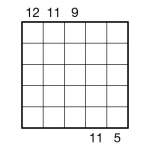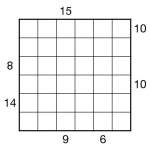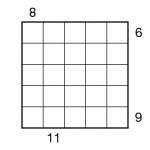# Sum Skyscraper

## Sum SkyscraperSum-Skyscraper 5×5 puzzle
I made this puzzle just before Christmas, and it’s been waiting on my desktop to be posted here ever since! Well, now it finally has been.This is a Sum Skyscraper. Place the digits 1 to 5 once each into every row and column in the grid. Numbers outside the grid provide the total (i.e. sum) of ‘visible’ grid digits along that row or column, if you imagine each digit as a building of that many storeys. Taller buildings always obscure shorter ones. So, for example, a clue for 21354 from the top of such a column would be 10, since the 2, 3 and 5 are visible (the 1 and 4 are obscured by the 2 and 5 respectively), and 2+3+5 = 10.

## Sum Skyscraper puzzles

Following-up yesterday’s Skyscraper puzzles, I thought I’d post a couple of Sum Skyscraper variant puzzles.Sum Skyscraper 6×6 puzzleSum Skyscraper 5×5 puzzle

Sum Skyscraper puzzles are very similar to Skyscraper puzzles, so no number can repeat in any row or column and external ’skyscraper’ clues reveal information about the numbers in the main grid. In 5×5 puzzles place 1-5, and in 6×6 puzzles place 1-6.

Each number in the completed grid represents a building of that many storeys, and place the buildings in such a way that each given number outside the grid represents the sum of the number of buildings that can be seen from that point, looking only at that number’s row or column. A building with a higher value always obscures a building with a lower value, while a building with a lower value never obscures a building with a higher value. So the clue ‘6′ in a 5×5 puzzle would indicate that the buildings ‘1′ and ‘5′ can be seen (’5′ is always visible in 5×5 puzzles), so the solution to a row might be 15234.